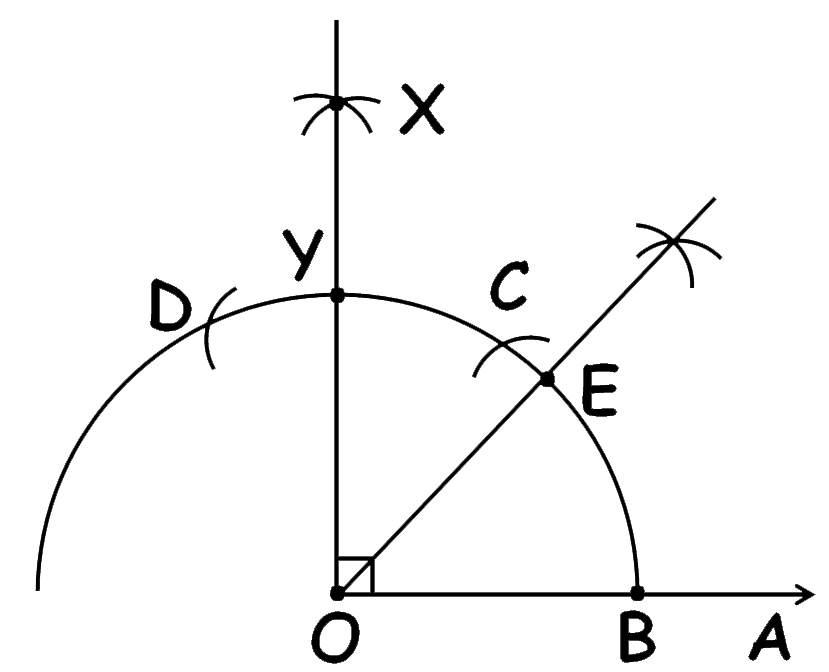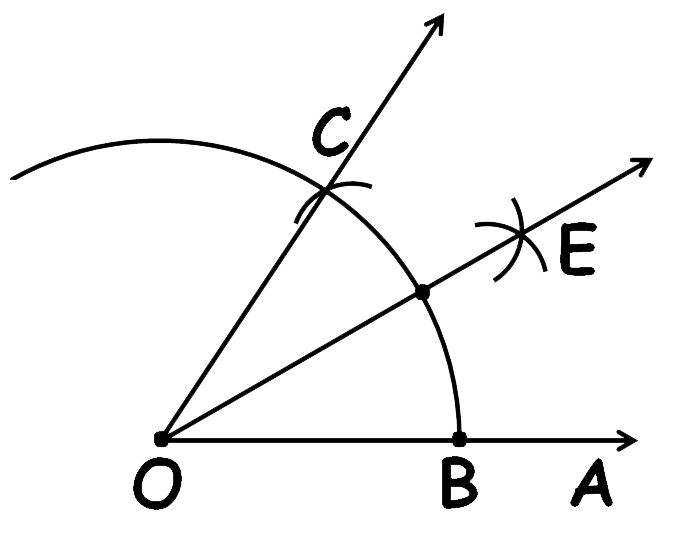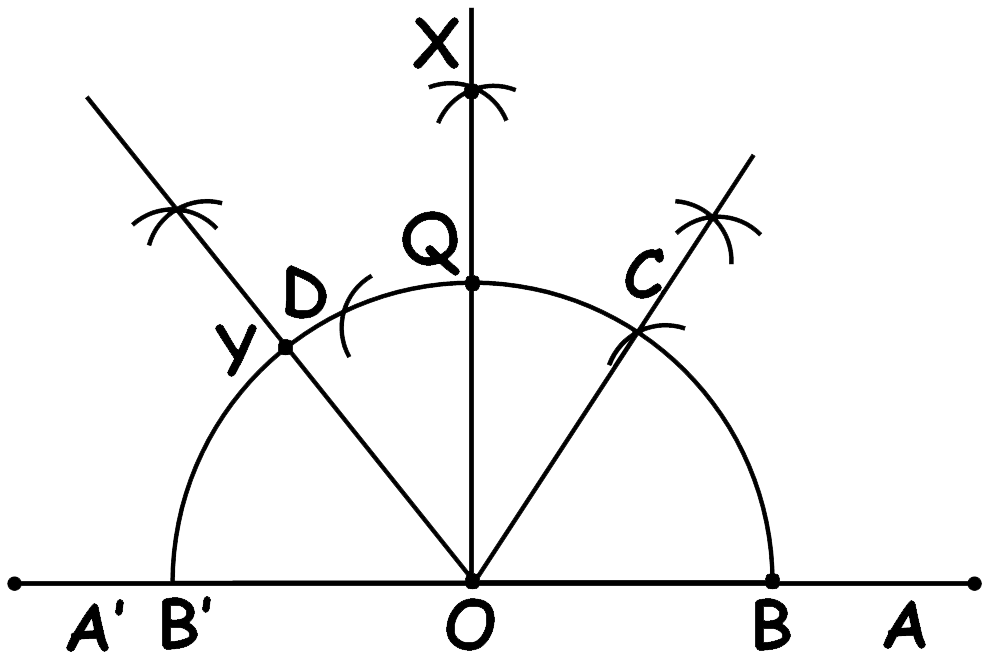# vs.eyeandcontacts.com

## Chapter 11 Constructions Exercise 11.1

Question 1: Construct an angle of 90° at the initial point of a given ray and justify the construction.

Construction steps:
1. Draw a ray OA.
2. With O as centre and any radius, draw a semicircle that cuts ray OA at B.
3. With B as centre and same radius, draw an arc to cut the semicircle at C.
4. With C as centre and same radius, draw an arc to cut the semicircle at D.
5. With C and D as centres and same radius, draw two arcs which intersect each other at X.
6. Finally, join XO which makes an angle of 90°.Justification
To justify that ∠XOA = 90°, we have to join OC and OD.
We have, ∠COA = ∠DOC = 60°. From the fourth and fifth step of this construction, we know that OX is the bisector of ∠DOC.
∴ ∠XOC = 1/2 of ∠DOC
= ∠XOC = 1/2 x 60° = 30°

Also, ∠XOA = ∠COA + ∠XOC
= 60° + 30° = 90°
Hence justified.

Question 2: Construct an angle of 45° at the initial point of a given ray and justify the construction.

Construction steps:
1. Draw a ray OA.
2. With O as centre and any radius, draw a semicircle that cuts OA at B.
3. With B as centre and same radius, draw an arc to cut the semicircle at C.
4. With C as centre and same radius, draw an arc to cut the semicircle at D.
5. With C and D as centres and same radius, draw two arcs which intersect each other at X.
6. Join XO to intersect the semicircle at Y, which makes an angle 90°.
7. Now, with Y and B as centres and radius more than half of YB draw intersecting arcs to meet at E.
8. Join OE. Therefore ∠EOA = 45°.Justification
From the construction,
∠XOA = 90°
As EO is the perpendicular bisector of ∠XOA. So,
∠EOA = 1/2 (∠XOA)
= ∠EOA = 1/2 × 90° = 45°.
Hence justified.

Question 3: Construct the angles of the following measurements:
i) 30°
ii) 22½°
iii) 15°

(i) 30°
Construction steps:
1. Draw a ray OA.
2. Taking O as a centre and any radius, draw an arc that cuts ray OA at B.
3. Now with B as centre and same radius draw an arc on the previous arc to cut it at C. This will be 60°.
4. With B and C as centres and radius more than half of BC, draw 2 arcs which intersect each other at E.
5. Join EO. Thus, ∠EOA = 30°.ii) 22½°
Construction steps:
1. Draw a ray OA.
2. With O as centre and any radius, draw a arc that cuts OA at B.
3. With B as centre and same radius, draw an arc to cut the semicircle at C.
4. With C as centre and same radius, draw an arc to cut the semicircle at D.
5. With C and D as centres and same radius, draw two arcs which intersect each other at X.
6. Join XO to intersect the semicircle at Y, which makes an angle of 90°.
7. Now, with Y and B as centres and radius more than half of YB draw intersecting arcs to meet at E.
8. Join OE. Therefore ∠EOA = 45°.
9. Finally, with E and B as centres and radius more than the half of BE draw 2 intersecting arcs to meet at F.
10. Join FO. Therefore ∠FOA = 22½°.iii) 15°
Construction steps:
1. Draw a ray OA.
2. Taking O as a centre and any radius, draw a semicircle that cuts ray OA at B.
3. Now with B as centre and same radius draw an arc on the semicircle to cut it at C. This will be 60°.
4. With B and C as centres and radius more than half of BC, draw 2 arcs which intersect each other at E.
5. Join EO to intersect the arc at F. Thus, ∠EOA = 30°.
6. With F and B as centres and radius more than the half of FB, draw 2 intersecting arcs to intersect at D. Join DO. Thus, ∠DOA = 15°.Question 4: Construct the following angles and verify by measuring them by a protractor:
i) 75°
ii) 105°
iii) 135°

i) 75°
Construction steps:
1. Draw a ray OA.
2. With O as centre and any radius draw an arc to intersect the ray OA at B.
3. With B as centre and same radius draw an arc to cut the semicircle at C.
4. With C as centre and same radius draw an arc to cut the semicircle at D.
5. With D and C as centre and same radius draw 2 arcs to intersect at X. Join OX. Let this line intersect the arc at Q.
6. With Q and C as centre draw 2 arcs to intersect at Y.
7. Join OY. Thus, ∠YOA = 75°.ii) 105°
Construction steps:
1. Draw a ray OA.
2. With O as centre and any radius draw a semicircle to cut ray OA at B.
3. With B as centre and same radius draw an arc to cut the semicircle at C.
4. With C as centre and same radius draw an arc on the arc to cut at D.
5. With D and C as centre and same radius draw 2 arcs to intersect at X. Join OX. Let this line intersect the semicircle at Q.
6. With Q and D as centre draw 2 arcs to intersect at Y.
7. Join OY. Thus, ∠YOA = 105°.iii) 135°
Construction steps:
1. Draw a line A’OA.
2. With O as centre and any radius draw a semicircle to intersect the line A’OA at B and B’.
3. With B as centre and same radius draw an arc to cut the semicircle at C.
4. With C as centre and same radius draw an arc to cut the semicircle at D.
4. With D and C as centre and same radius draw 2 arcs to intersect at X. Join OX. Let this line intersect the semicircle at Q.
5. With Q and B’ as centre draw 2 arcs to intersect at Y.
6. Join OY. Thus, ∠YOA = 135°.Question 5: Construct an equilateral triangle, given its side and justify the construction.

Construction steps:
1. Draw a line segment, AB of any units (say 5cm).
2. With A and B as centres, draw two semicircle on the line segment AB to cut it at D and E respectively.
3. With D and E as centres, draw 2 arcs that cuts the semicircle at G and F respectively to form an angle of 60° each.
4. Now, join AG and BF and extend such that they meet each other at the point C.
5. Therefore, ABC is the required triangle.Justification:
After construction we observe
AB = 5 cm, ∠A = 60° and ∠B = 60°
We know that the sum of the interior angles of a triangle is 180°
∴ ∠A + ∠B + ∠C = 180°
= 60° + 60° + ∠C = 180° (as ∠A and ∠B are 60° each)
= 120° + ∠C = 180°
= ∠C = 60°
We have one side equal. To prove that other 2 sides are also equal,
∴ BC = CA = 5 cm (Sides opposite to equal angles are equal)
AB = BC = CA = 5 cm
∠A = ∠B = ∠C = 60°
Hence, justified.